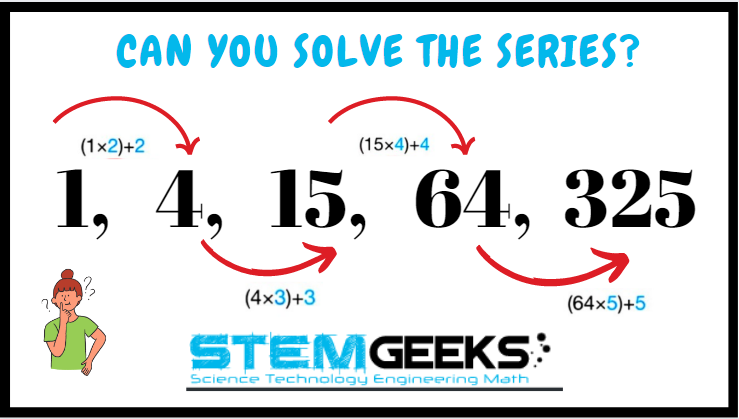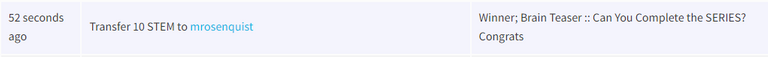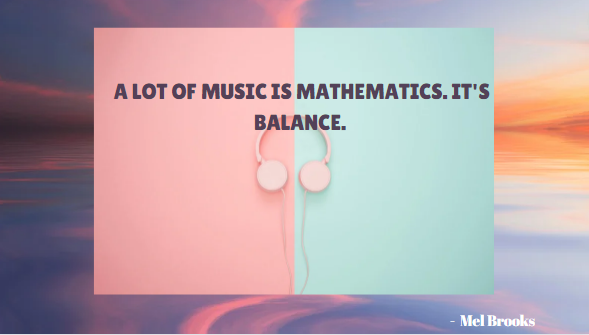# Brain Teaser :: Can You Solve the EQUATION?

in STEMGeeks3 months ago

Hey All; @StemGeeks Mathematician;

Without further, ado, let's get to our Brain Teaser :: Can You Complete the SERIES?Have a close look at the image given and see if you can solve the simple tricky equation. This is not that difficult equation to solve and I assure that it should be FUN to solve this EQUATION... Just remember there hasn't have to be a logic always.

There are few different solution and simple basic math's knowledge is required to get the equation right. Just give it a try - all you need to do is pick up a rough paper and see the equation that's being formed and you will get the answer. Try it out it should be FUN and its that simple I assure you. There is no right or wrong - comment your answer on the reply box below to enter a chance to WIN 10 STEM tokens...

### STEM token GiveAway...

I'll be again doing a giveaway of STEM tokens to the lucky random winner with the correct answer. For the last contest, which was Brain Teaser :: Can You Complete the SERIES?.### Solution :: Brain Teaser :: Can You Complete the SERIES?

###### Here we had to apply the Formula --> First number * 2 + 2 followed by Second Number * 3 + 3 and so forth; lets look at how we solve the series

- ( 1 * 2 ) + 2 = 4

- ( 4 * 3 ) + 3 = 15- ( 15 * 4 ) + 4 = 64

- ( 64 * 5 ) + 5 = 325

Finally the answer to the puzzle was:: 325

I guess this was a tuff problem to solve and we had just two entries for the same. And both of the entries provided the right the right answer.. I am going to use a random picker tool to pick a random winner.Congratulations @mrosenquist - You WIN yourself 10 STEM tokens each. You should be having the rewards in your STEM Wallet Soon.

### Math Quote for the Day::

Here is the motivation to solve the Math Puzzle?If you like my work, then please spread the Word.. that we do have the Math Brain Teasers competition here @StemGeeks platform. Reblog is much appreciated.

Best RegardsFind Me on the Other Social Media Platforms::PS:: All the Maths Brain Teasers; are made by me using the Pro Canva License Version

Sort:

I'm going with 4, according to "please excuse my dear aunt Sally"

Following BODMAS rule the answer is 4

die Antwort ist 4

da 3 - (3*0) + (3/3) = 4

The answer is 4, using bodmas
3 - (3*0) + (3/3) = 3-0+1 = 4

Congratulations, @gungunkrishu Your Post Got 82.5% Boost.
@gungunkrishu Burnt 28 UPME & We Followed That Lead.